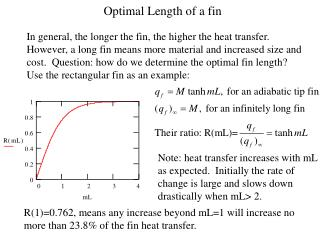Download PresentationOptimal Length of a fin

# Optimal Length of a fin - PowerPoint PPT PresentationDownload Presentation## Optimal Length of a fin

- - - - - - - - - - - - - - - - - - - - - - - - - - - E N D - - - - - - - - - - - - - - - - - - - - - - - - - - -
##### Presentation Transcript

1. Optimal Length of a fin In general, the longer the fin, the higher the heat transfer. However, a long fin means more material and increased size and cost. Question: how do we determine the optimal fin length? Use the rectangular fin as an example: Note: heat transfer increases with mL as expected. Initially the rate of change is large and slows down drastically when mL> 2. R(1)=0.762, means any increase beyond mL=1 will increase no more than 23.8% of the fin heat transfer.

2. Temperature Distribution • Use m=5, and L=0.2 as an example: Low DT, poor fin heat transfer High DT, good fin heat transfer

3. Correction Length for a Fin with a Non-adiabatic Tip • The correction length can be determined by using the formula: Lc=L+(Ac/P), where Ac is the cross-sectional area and P is the perimeter of the fin at the tip. • Thin rectangular fin: Ac=Wt, P=2(W+t)2W, since t < W Lc=L+(Ac/P)=L+(Wt/2W)=L+(t/2) • Cylindrical fin: Ac=(/4)D2, P= D, Lc=L+(Ac/P)=L+(D/4) • Square fin: Ac=W2, P=4W, Lc=L+(Ac/P)=L+(W2/4W)=L+(W/4)